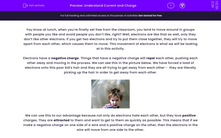# Understand Current and Charge

In this worksheet, students will explore what electrical current is and how it flows, using the idea of charge.Key stage:  KS 4

Year:  GCSE

GCSE Boards:   AQA, AQA Trilogy, AQA Synergy, OCR 21st Century, OCR Gateway, Pearson Edexcel, Eduqas,

Popular topics:   Physics worksheets

Difficulty level:#### Worksheet Overview

You know at lunch, when you’re finally set free from the classroom, you tend to move around in groups with people you like and avoid people you don’t like, right? Well, electrons are like that as well, only they don’t like other electrons. If you get two electrons and try to put them close together, they will try to move apart from each other, which causes them to move. This movement of electrons is what we will be looking at in this activity.

Electrons have a negative charge. Things that have a negative charge will repel each other, pushing each other away and moving in the process. We can see this in the picture below. We have forced a load of electrons onto this poor kid’s hair and they are all trying to get away from each other -  they are literally picking up the hair in order to get away from each other.We can use this to our advantage because not only do electrons hate each other, but they love positive charges. They are attracted to them and want to get to them as quickly as possible. This means that if we make a negative charge on one side of a wire and a positive charge on the other, then the electrons in the wire will move from one side to the other.

Why is this so important? It is all because of the definition of current. An electric current is a flow of electric charge, carried by negatively charged electrons in the atoms of metals.

Now for some maths! We can work out the current by using the following equation:

I = Q ÷ t

current = charge divided by time

I = current measured in amperes (A)

Q = charge measured in coulombs (C)

t = time measured in seconds (s)

You will have to remember this equation!

Let's go through an example of how to use it:

Let's imagine that a charge of 3 C passes through a wire every second. What is the current in the wire?

Step 1   Find and highlight all of the numbers.

A charge of 3 C passes through a wire every second. What is the current in the wire?

Step 2   Write out the numbers next to their symbols

I = ?

Q = 3 C

t = 1 s

Step 3   Put them into the equation I = Q ÷ t

I = 3 ÷ 1

Step 4  Put this into your calculator and work out the answer and don’t forget the units!

I = 3 A

Now, let's have a go at some questions.

### What is EdPlace?

We're your National Curriculum aligned online education content provider helping each child succeed in English, maths and science from year 1 to GCSE. With an EdPlace account you’ll be able to track and measure progress, helping each child achieve their best. We build confidence and attainment by personalising each child’s learning at a level that suits them.

Get started